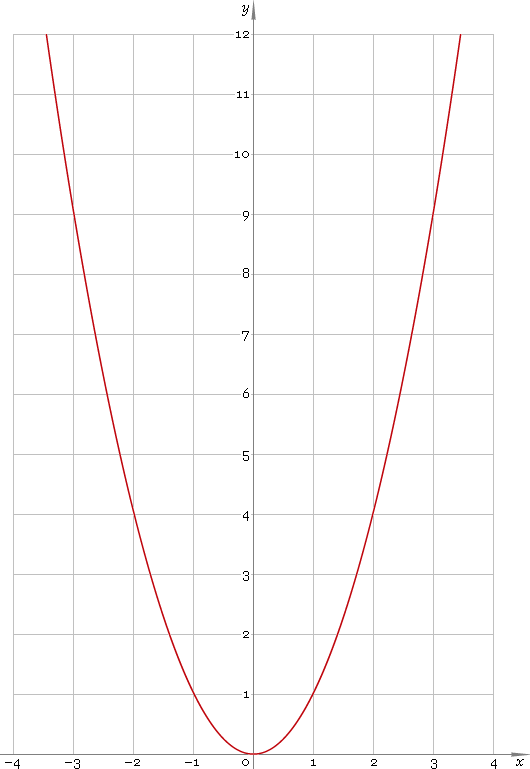The Art of Interface

# ^ — power function

Category. Mathematics.

Abstract. Power: definition, plot, properties and identities.

## 1. Definition

Power function is the function of kind

xa

## 2. Plot

Power function domain depends on power a, but it is always defined for positive real half-axis (0, +∞). Parabola plot for a = 2 is depicted below — fig. 1.Fig. 1. Plot of the power function y = x2.

Function codomain as well depends on power a, but it always includes positive half of the real axis (0, +∞).

## 3. Identities

Negative power:

xa = 1 /xa

Power sum and difference:

xa + b = xa xb
xab = xa/xb

Power product:

xab = (xa)b

## 4. Support

Power function ^ of the real argument is supported by free version of the Librow calculator.

Power function ^ of the complex argument is supported by professional version of the Librow calculator.

## 5. How to use

To calculate power of the number:

``9^2;``

To calculate power of the current result:

``rslt^2;``

To engage the current result as power:

``9^rslt;``

To calculate power of the number x in memory:

``mem[x]^2;``

To engage number x in memory as power:

``9^mem[x];``